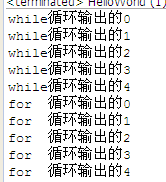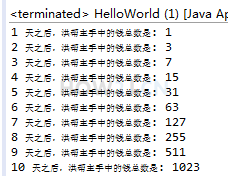控制流程系列教材 （三）- java的while语句 控制流程系列教材 （五）- java 的 continue语句

for循环，和while一样，只是表达方式不一样4分9秒

## forpublic class HelloWorld { public static void main(String[] args) { //使用while打印0到4 int i = 0; while(i<5){ System.out.println("while循环输出的"+i); i++; } //使用for打印0到4 for (int j = 0; j < 5; j++) { System.out.println("for 循环输出的"+j); } } }
```public class HelloWorld {
public static void main(String[] args) {

//使用while打印0到4
int i = 0;
while(i<5){
System.out.println("while循环输出的"+i);
i++;
}

//使用for打印0到4
for (int j = 0; j < 5; j++) {
System.out.println("for  循环输出的"+j);
}
}
}
```

## 练习-乞丐

姿势不对,事倍功半! 点击查看做练习的正确姿势

## 答案-乞丐4分44秒 本视频采用html5方式播放，如无法正常播放，请将浏览器升级至最新版本，推荐火狐，chrome，360浏览器。 如果装有迅雷，播放视频呈现直接下载状态，请调整 迅雷系统设置-基本设置-启动-监视全部浏览器 (去掉这个选项)。 chrome 的 视频下载插件会影响播放，如 IDM 等，请关闭或者切换其他浏览器public class HelloWorld { public static void main(String[] args) { int moneyEachDay = 0; int day = 10; int sum=0; for (int i = 1; i <= day; i++) { if(0==moneyEachDay) moneyEachDay = 1; else moneyEachDay *= 2; sum+=moneyEachDay; System.out.println(i + " 天之后，洪帮主手中的钱总数是: " + sum ); } } }
```public class HelloWorld {
public static void main(String[] args) {

int moneyEachDay = 0;
int day = 10;
int sum=0;
for (int i = 1; i <= day; i++) {
if(0==moneyEachDay)
moneyEachDay = 1;
else
moneyEachDay *= 2;

sum+=moneyEachDay;

System.out.println(i + " 天之后，洪帮主手中的钱总数是: " + sum );
}
}

}
```

 控制流程系列教材 （三）- java的while语句 控制流程系列教材 （五）- java 的 continue语句

HOW2J公众号，关注后实时获知最新的教程和优惠活动，谢谢。2021-01-14 这才是最简单的方法吧，哈哈
 qq519509989 关于 JAVA 基础-控制流程-for 的提问 提示：运用位运算 ```import java.util.Scanner; public class HelloWorld { public static void main(String[] args) { int result = 0; /*while (i<=10) { result += 1 << (i-1); i++; }*/ for (int j = 1; j <= 10; j++) { result += 1 << (j - 1); } System.out.println(result); } }```

3 个答案

Java_learner66

public class Test{ public static void main(String[] args) { int sum=0; int a=2; for(int i=1;i<=10;i++) { sum += Math.pow(a, i-1); } System.out.print(sum); } }

slw123

Kore

int sum = 0; int money = 1; for (int i = 1;i <= 10;i++) { sum += money; money *= 2; } System.out.println("10天乞丐收入为：" + sum);

2021-01-08 这样
 环保people 关于 JAVA 基础-控制流程-for 的提问 这样就行 ```public class Test04 { public static void main(String[] args) { int sum = 0; for(int i = 1 ; i<=10 ;i++){ sum += (int) Math.pow(2,i-1); } System.out.println("乞丐干十天收入是"+sum+"元"); } }```

2021-01-08 参考
2021-01-06 这样可以吗
2020-12-08 等比数列求和，很简洁的方法